# RD Sharma Class 9 Solutions Chapter 5 Factorisation of Algebraic Expressions Ex 5.4

## RD Sharma Class 9 Solutions Chapter 5 Factorisation of Algebraic Expressions Ex 5.4

These Solutions are part of RD Sharma Class 9 Solutions. Here we have given RD Sharma Class 9 Solutions Chapter 5 Factorisation of Algebraic Expressions Ex 5.4

Other Exercises

Factorize each of the following expressions:
Question 1.
a3 + 8b3 + 64c3 – 24abc
Solution:
We know that
a3 + b3 + c3 – 3abc = (a + b + c) (a2 + b2 + c2 – ab – bc – ca)
a3 + 8b3 + 64c3 – 24abc
= (a)3 + (2b)3 + (4c)3 – 3 x a x 2b x 4c
= (a + 2b + 4c) [(a)2 + (2b)2 + (4c)2 -a x 2b – 2b x 4c – 4c x a]
= (a + 2b + 4c) (a2 + 4b2 + 16c2 – 2ab – 8bc – 4ca)

Question 2.
x3 – 8y3 + 27z3 + 18xyz
Solution:
x3 – 8y3 + 27z3 + 18xyz
= (x)3 + (-2y)3 + (3z)3 – 3 x x x (-2y) (3 z)
= (x – y + 3z) (x2 + 4y2 + 9z2 + 2xy + 6yz – 3zx)

Question 3.
27x3 – y3 – z3 – 9xyz          [NCERT]
Solution:
27x3-y3-z3-9xyz
= (3x)3 + (-y)3 + (-z)3 – 3 x 3x x (-y) (-z)
= (3x – y – z) [(3x)2 + (-y)2 + (-z)2 – 3x x (-y) – (-y) (-z)-  (- z x 3x)]
= (3x-y – z) (9x2y2 + z2 + 3xy – yz + 3zx)

Question 4.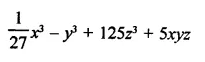Solution: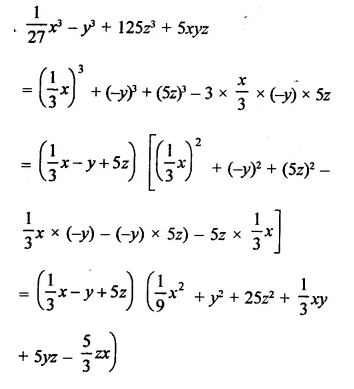Question 5.
8x3 + 27y3 – 216z3 + 108xyz
Solution:
8x3 + 27y3 – 216z3 + 108xyz
= (2x)3 + (3y)3 + (6z)3 – 3 x (2x) (3y) (-6z)
= (2x + 3y – 6z) [(2x)2 + (3y)2 + (-6z)2 – 2x x 3y – 3y x (-6z) – (-6z) x 2x]
= (2x + 3y – 6z) (4x2 + 92 + 36z2 – 6xy + 18yz + 12zx)

Question 6.
125 + 8x3 – 27y3 + 90xy
Solution:
125 + 8X3 – 27y3 + 90xy
= (5)3 + (2x)3 + (-3y)3 – [3 x 5 x 2x x (-3y)]
= (5 + 2x – 3y) [(5)2 + (2x)2 + (-3y)2 – 5 x 2x – 2x (-3y) – (-3y) x 5]
= (5 + 2x – 3y) (25 + 4x2 + 9y2– 10x + 6xy + 15y)

Question 7.
8x3 – 125y3 + 180xy + 216
Solution:
8x3 – 125y3 + 180xy + 216
= (2x)3 + (-5y)3 + (6)3 – 3 x 2x (-5y) x 6
= (2x – 5y + 6) [(2x)2 + (-5y)2 + (6)2 – 2x x (-5y) – (-5y) x 6 – 6 x 2x]
= (2x -5y + 6) (4x2 + 25y2 + 36 + 10xy + 30y – 12x)

Question 8.
Multiply:
(i) x2 +y2 + z2 – xy + xz + yz by x + y – z
(ii) x2 + 4y2 + z2 + 2xy + xz – 2yz by x- 2y-z
(iii) x2 + 4y2 + 2xy – 3x + 6y + 9 by x – 2y + 3
(iv) 9x2 + 25y2 + 15xy + 12x – 20y + 16 by 3x  – 5y + 4
Solution:
(i)  (x2 + y2 + z2 – xy + yz + zx) by (x + y – z)
= x3 +y3 – z3 + 3xyz
(ii) (x2 + 4y2 + z2 + 2xy + xz – 2yz) by (x – 2y – z)
= (x -2y-z) [x2 + (-2y)2 + (-z)2 -x x (- 2y) – (-2y) (z) – (-z) (x)]
= x3 + (-2y)3 + (-z)3 – 3x (-2y) (-z)
= x3 – 8y3 – z3 – 6xyz
(iii) x2 + 4y2 + 2xy – 3x + 6y + 9 by x – 2y + 3
= (x – 2y + 3) (x2 + 4y2 + 9 + 2xy + 6y – 3x)
= (x)3 + (-2y)3 + (3)3 – 3 x x x (-2y) x 3 = x3 – 8y3 + 27 + 18xy
(iv) 9x2 + 25y3 + 15xy + 12x – 20y + 16 by 3x – 5y + 4
= (3x -5y + 4) [(3x)2 + (-5y)2 + (4)2 – 3x x (-5y) (-5y x 4) – (4 x 3x)]
= (3x)3 + (-5y)3 + (4)3 – 3 x 3x (-5y) x 4
= 27x3 – 12573 + 64 + 180xy

Question 9.
(3x – 2y)3 + (2y – 4z)3 + (4z – 3x)3
Solution:
(3x – 2y)3 + (2y – 4z)+   (4z – 3x)3
∵ 3x – 2y + 2y – 4z + 4z – 3x = 0
∴ (3x – 2y)3 + (2y – 4z)3 + (4z – 3x)3
= 3(3x – 2y) (2y – 4z) (4z – 3x)               {∵ x3 + y3 + z3 = 3xyz if x + y + z = 0}

Question 10.
(2x – 3y)3 + (4z – 2x)3  + (3y – 4z)3
Solution:
(2x – 3y)3 + (4z – 2x)3 + (3y – 4z)3
∵  2x – 3y + 4z – 2x + 3y – 4z = 0
∴ (2x – 3y)3 + (4z – 2x)3 + (3y – 4z)3
= (2x – 3y) (4z – 2x) (3y – 4z)                {∵ x3 + y3 + z3 = 3xyz if x + y + z = 0}

Question 11.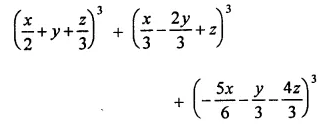Solution: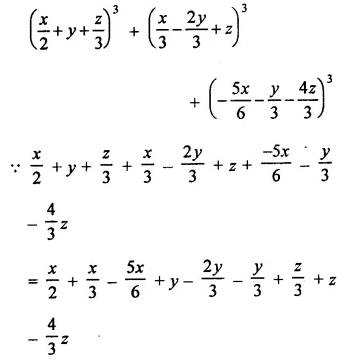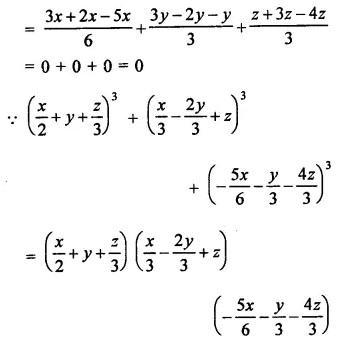Question 12.
(a – 3b)3 + (3b – c)3 + (c – a)3
Solution:
(a- 3b)3 + (3b – c)3 + (c – a)3
∵ a – 3b + 3b – c + c – a = 0
∴  (a – 3b)3 + (3b – c)3 + (c – a)3
= 3(a – 3b) (3b – c) (c – a)                       {∵ a3 + b3 + c3 = 3abc if a + b + c = 0}

Question 13.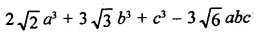Solution: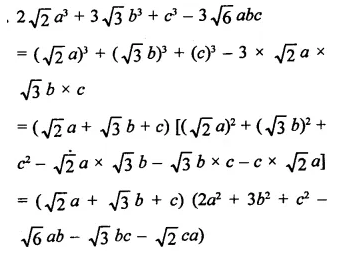Question 14.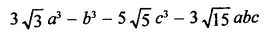Solution: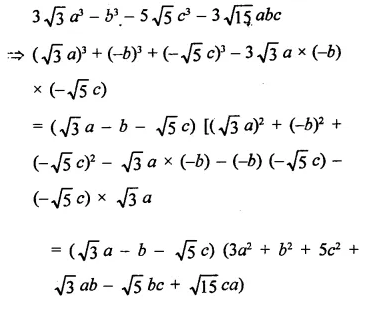Question 15.
2 $$\sqrt { 2 }$$ a3+ 16$$\sqrt { 2 }$$ b3 + c3 – 12abc
Solution: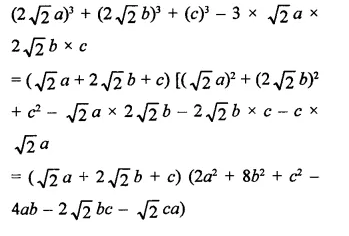Question 16.
Find the value of x3 + y– 12xy + 64, when x + y = -4
Solution:
x3 + y– 12xy + 64
x + y = -4
Cubing both sides,
x3 + y3 + 3 xy(x + y) = -64
Substitute the value of (x + y)
⇒ x2 + y2 + 3xy x (-4) = -64
⇒  x3 + y2 – 12xy + 64 = 0

Hope given RD Sharma Class 9 Solutions Chapter 5 Factorisation of Algebraic Expressions Ex 5.4 are helpful to complete your math homework.

If you have any doubts, please comment below. Learn Insta try to provide online math tutoring for you.